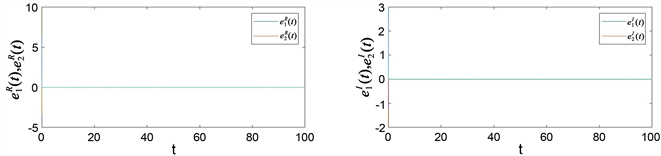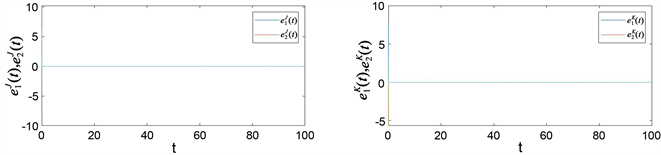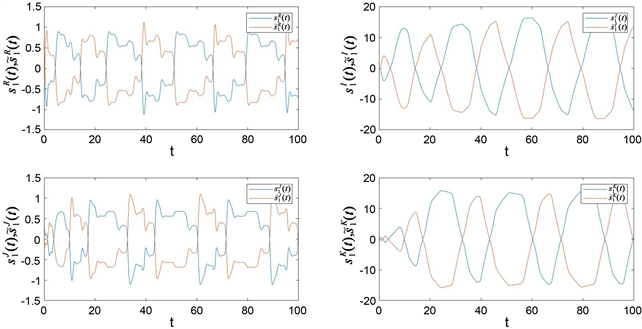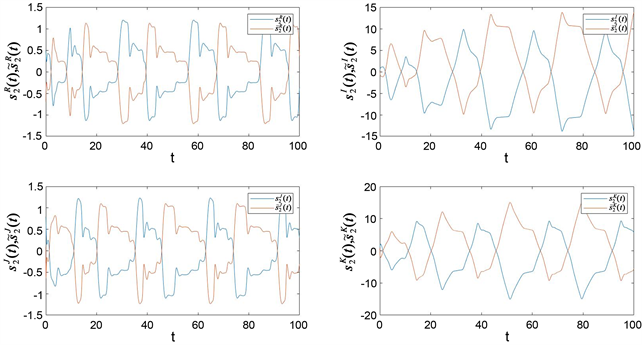﻿ 一类带时滞的四元数神经网络的全局指数反同步

# 一类带时滞的四元数神经网络的全局指数反同步Global Exponential Anti-Synchronization for a Class of Quaternion-Valued Neural Networks with Time Delays

Abstract: In this paper, the problem of global exponential anti-synchronization for a class of quaterni-on-valued neural networks (QVNNs) with time delays is studied. Firstly, in light of Hamilton’s law, the QVNNs were decomposed into four real-valued parts to compose the equivalent real-valued sys-tems. Next, by constructing appropriate Lyapunov-Krasovskii functionals, utilizing drive-response synchronization method and matrix inequality theory, a result about global exponential an-ti-synchronization of QVNNs with time delays is realized. Finally, a numerical example was given to verify the effectiveness of the proposed method.

1. 引言

2. 预备知识

2.1. 四元数代数

$q={q}_{1}+{q}_{2}i+{q}_{3}j+{q}_{4}k,$

${i}^{2}={j}^{2}={k}^{2}=ijk=-1,\text{}ij=-ji=k,\text{}jk=-kj=i,\text{}ki=-ik=j,$

${q}_{1}$ 为四元数 $q$ 的实部，记为 $Re\left(q\right)={q}_{1}$${q}_{2}i+{q}_{3}j+{q}_{4}k$ 称为四元数 $q$ 的虚部，记为 $Im\left(q\right)={q}_{2}i+{q}_{3}$ $j+{q}_{4}k$。由此我们可知四元数乘法不可交换。定义另外一个四元数

$p={p}_{1}+{p}_{2}i+{p}_{3}j+{p}_{4}k,$

$p+q=\left({p}_{1}+{q}_{1}\right)+\left({p}_{2}+{q}_{2}\right)i+\left({p}_{3}+{q}_{3}\right)j+\left({p}_{4}+{q}_{4}\right)k,$

$\begin{array}{l}pq=\left({p}_{1}{q}_{1}-{p}_{2}{q}_{2}-{p}_{3}{q}_{3}-{p}_{4}{q}_{4}\right)+\left({p}_{1}{q}_{2}+{p}_{2}{q}_{1}+{p}_{3}{q}_{4}-{p}_{4}{q}_{3}\right)i+\left({p}_{1}{q}_{3}+{p}_{3}{q}_{1}+{p}_{4}{q}_{2}-{p}_{2}{q}_{4}\right)j\\ \text{}+\left({p}_{1}{q}_{4}+{p}_{4}{q}_{1}+{p}_{2}{q}_{3}-{p}_{3}{q}_{2}\right)k.\end{array}$

${p}^{\ast }=\stackrel{¯}{p}={p}_{1}-{p}_{2}i-{p}_{3}j-{p}_{4}k$ 表示 $p$ 的共轭转置，并且 $p$ 的模表示为

$|p|=\sqrt{p{p}^{*}}=\sqrt{{p}_{1}^{2}+{p}_{2}^{2}+{p}_{3}^{2}+{p}_{4}^{2}}.$

$u={\left({u}_{1},{u}_{2},\cdots ,{u}_{n}\right)}^{\text{T}}\in {ℚ}^{n}$，则 $u$ 的模可表示为 $|u|={\left(|{u}_{1}|,|{u}_{2}|,\cdots ,|{u}_{n}|\right)}^{\text{T}}$$u$ 的范数表示为

$‖u‖\text{=}\sqrt{\underset{i=1}{\overset{n}{\sum }}{|{u}_{i}|}^{2}}.$

2.2. 系统表示及基本引理

$\stackrel{˙}{s}\left(t\right)=-As\left(t\right)+Bf\left(s\left(t\right)\right)+Cg\left(s\left(t-\tau \right)\right)+J,$ (1)

$\stackrel{˙}{\stackrel{˜}{s}}\left(t\right)=-A\stackrel{˜}{s}\left(t\right)+Bf\left(\stackrel{˜}{s}\left(t\right)\right)+Cg\left(\stackrel{˜}{s}\left(t-\tau \right)\right)+J+U\left(t\right),$ (2)

$f\left(x\right)={f}^{R}\left({x}^{R}\right)+{f}^{I}\left({x}^{I}\right)i+{f}^{J}\left({x}^{J}\right)j+{f}^{K}\left({x}^{K}\right)k,$

$g\left(x\right)={g}^{R}\left({x}^{R}\right)+{g}^{I}\left({x}^{I}\right)i+{g}^{J}\left({x}^{J}\right)j+{g}^{K}\left({x}^{K}\right)k,$

$\begin{array}{l}|{f}_{p}^{\left(\mu \right)}\left({s}_{1}^{\left(\mu \right)}\right)+{f}_{p}^{\left(\mu \right)}\left({s}_{2}^{\left(\mu \right)}\right)|\le {l}_{p}^{\left(\mu \right)}|{s}_{1}^{\left(\mu \right)}+{s}_{2}^{\left(\mu \right)}|,\\ |{g}_{p}^{\left(\mu \right)}\left({s}_{1}^{\left(\mu \right)}\right)+{g}_{p}^{\left(\mu \right)}\left({s}_{2}^{\left(\mu \right)}\right)|\le {m}_{p}^{\left(\mu \right)}|{s}_{1}^{\left(\mu \right)}+{s}_{2}^{\left(\mu \right)}|,\end{array}$ (3)

${D}^{+}p\left(t\right)\le -{k}_{1}p\left(t\right)+{k}_{2}\stackrel{˜}{p}\left(t\right),$

$p\left(t\right)\le \text{}\stackrel{˜}{p}\left({t}_{0}\right){e}^{-\lambda \left(t-{t}_{0}\right)},\text{}t\ge {t}_{0},$

$2{x}^{\text{T}}{\text{Ω}}_{1}^{\text{T}}{\text{Ω}}_{2}y\le {x}^{\text{T}}{\text{Ω}}_{1}^{\text{T}}{\text{Ω}}_{3}{\text{Ω}}_{1}x+{y}^{\text{T}}{\text{Ω}}_{2}^{\text{T}}{\text{Ω}}_{3}^{-1}{\text{Ω}}_{2}y$

$\stackrel{˙}{e}\left(t\right)=-Ae\left(t\right)+B\stackrel{˜}{f}\left(e\left(t\right)\right)+C\stackrel{˜}{g}\left(e\left(t-\tau \left(t\right)\right)\right)+U\left(t\right),$ (4)

$‖e\left(t\right)‖\le M\underset{-\tau \le s\le 0}{\mathrm{sup}}‖e\left(s\right)‖\mathrm{exp}\left(-\kappa t\right),$

$\begin{array}{l}{\stackrel{˙}{e}}^{R}\left(t\right)=-A{e}^{R}+{B}^{R}{\stackrel{˜}{f}}^{R}\left({e}^{R}\left(t\right)\right)-{B}^{I}{\stackrel{˜}{f}}^{I}\left({e}^{I}\left(t\right)\right)-{B}^{J}{\stackrel{˜}{f}}^{J}\left({e}^{J}\left(t\right)\right)-{B}^{K}{\stackrel{˜}{f}}^{K}\left({e}^{K}\left(t\right)\right)+{C}^{R}{\stackrel{˜}{g}}^{R}\left({e}^{R}\left(t-\tau \right)\right)\\ \text{}-{C}^{I}{\stackrel{˜}{g}}^{I}\left({e}^{I}\left(t-\tau \right)\right)-{C}^{J}{\stackrel{˜}{g}}^{J}\left({e}^{J}\left(t-\tau \right)\right)-{C}^{K}{\stackrel{˜}{g}}^{K}\left({e}^{K}\left(t-\tau \right)\right)+{U}^{R}\left(t\right),\\ {\stackrel{˙}{e}}^{I}\left(t\right)=-A{e}^{I}+{B}^{R}{\stackrel{˜}{f}}^{I}\left({e}^{I}\left(t\right)\right)+{B}^{I}{\stackrel{˜}{f}}^{R}\left({e}^{R}\left(t\right)\right)+{B}^{J}{\stackrel{˜}{f}}^{K}\left({e}^{K}\left(t\right)\right)-{B}^{K}{\stackrel{˜}{f}}^{J}\left({e}^{J}\left(t\right)\right)+{C}^{R}{\stackrel{˜}{g}}^{I}\left({e}^{I}\left(t-\tau \right)\right)\\ \text{}+{C}^{I}{\stackrel{˜}{g}}^{R}\left({e}^{R}\left(t-\tau \right)\right)+{C}^{J}{\stackrel{˜}{g}}^{K}\left({e}^{K}\left(t-\tau \right)\right)-{C}^{K}{\stackrel{˜}{g}}^{J}\left({e}^{J}\left(t-\tau \right)\right)+{U}^{I}\left(t\right),\\ {\stackrel{˙}{e}}^{J}\left(t\right)=-A{e}^{J}+{B}^{R}{\stackrel{˜}{f}}^{J}\left({e}^{J}\left(t\right)\right)+{B}^{J}{\stackrel{˜}{f}}^{R}\left({e}^{R}\left(t\right)\right)-{B}^{I}{\stackrel{˜}{f}}^{K}\left({e}^{K}\left(t\right)\right)+{B}^{K}{\stackrel{˜}{f}}^{I}\left({e}^{I}\left(t\right)\right)+{C}^{R}{\stackrel{˜}{g}}^{J}\left({e}^{J}\left(t-\tau \right)\right)\\ \text{}+{C}^{J}{\stackrel{˜}{g}}^{R}\left({e}^{R}\left(t-\tau \right)\right)-{C}^{I}{\stackrel{˜}{g}}^{K}\left({e}^{K}\left(t-\tau \right)\right)+{C}^{K}{\stackrel{˜}{g}}^{I}\left({e}^{I}\left(t-\tau \right)\right)+{U}^{J}\left(t\right),\\ {\stackrel{˙}{e}}^{K}\left(t\right)=-A{e}^{K}+{B}^{R}{\stackrel{˜}{f}}^{K}\left({e}^{K}\left(t\right)\right)+{B}^{K}{\stackrel{˜}{f}}^{R}\left({e}^{R}\left(t\right)\right)+{B}^{I}{\stackrel{˜}{f}}^{J}\left({e}^{J}\left(t\right)\right)-{B}^{J}{\stackrel{˜}{f}}^{I}\left({e}^{I}\left(t\right)\right)+{C}^{R}{\stackrel{˜}{g}}^{K}\left({e}^{K}\left(t-\tau \right)\right)\\ \text{}+{C}^{K}{\stackrel{˜}{g}}^{R}\left({e}^{R}\left(t-\tau \right)\right)+{C}^{I}{\stackrel{˜}{g}}^{J}\left({e}^{J}\left(t-\tau \right)\right)-{C}^{J}{\stackrel{˜}{g}}^{I}\left({e}^{I}\left(t-\tau \right)\right)+{U}^{K}\left(t\right).\end{array}$ (5)

3. 主要结果

${U}^{u}\left(t\right)=-{\zeta }_{p}^{u}{e}_{p}^{u}\left(t\right),$ (6)

$\begin{array}{l}{\zeta }^{u}>{\lambda }_{\mathrm{max}}\left(\Psi +2{\stackrel{¯}{L}}^{u}\right),\\ \mathrm{min}\left\{{\zeta }^{u}-{\lambda }_{\mathrm{max}}\left(\Psi +2{\stackrel{¯}{L}}^{u}\right)\right\}>\mathrm{max}\left\{{\lambda }_{\mathrm{max}}\left({\stackrel{¯}{M}}^{R}\right),{\lambda }_{\mathrm{max}}\left({\stackrel{¯}{M}}^{I}\right),{\lambda }_{\mathrm{max}}\left({\stackrel{¯}{M}}^{J}\right),{\lambda }_{\mathrm{max}}\left({\stackrel{¯}{M}}^{K}\right)\right\}>0,\end{array}$ (7)

$\text{Ψ}=-A+\frac{1}{2}\left\{{B}^{R}{\left({B}^{R}\right)}^{\text{T}}+{B}^{I}{\left({B}^{I}\right)}^{\text{T}}+{B}^{J}{\left({B}^{J}\right)}^{\text{T}}+{B}^{K}{\left({B}^{K}\right)}^{\text{T}}+{C}^{R}{\left({C}^{R}\right)}^{\text{T}}+{C}^{I}{\left({C}^{I}\right)}^{\text{T}}+{C}^{J}{\left({C}^{J}\right)}^{\text{T}}+{C}^{K}{\left({C}^{K}\right)}^{\text{T}}\right\},$

$V\left(t\right)=\frac{1}{2}\left({\left({e}^{R}\left(t\right)\right)}^{\text{T}}{e}^{R}\left(t\right)+{\left({e}^{I}\left(t\right)\right)}^{\text{T}}{e}^{I}\left(t\right)+{\left({e}^{J}\left(t\right)\right)}^{\text{T}}{e}^{J}\left(t\right)+{\left({e}^{K}\left(t\right)\right)}^{\text{T}}{e}^{K}\left(t\right)\right)$ (8)

$\stackrel{˙}{V}\left(t\right)={\left[{\stackrel{˙}{e}}^{R}\left(t\right)\right]}^{\text{T}}{e}^{R}\left(t\right)+{\left[{\stackrel{˙}{e}}^{I}\left(t\right)\right]}^{\text{T}}{e}^{I}\left(t\right)+{\left[{\stackrel{˙}{e}}^{J}\left(t\right)\right]}^{\text{T}}{e}^{J}\left(t\right)+{\left[{\stackrel{˙}{e}}^{K}\left(t\right)\right]}^{\text{T}}{e}^{K}\left(t\right)$ (9)

$\begin{array}{l}{\left[{\stackrel{˜}{f}}^{\left(\mu \right)}\left({e}^{\mu }\left(t\right)\right)\right]}^{\text{T}}{\stackrel{˜}{f}}^{\left(\mu \right)}\left({e}^{\mu }\left(t\right)\right)\le {\left[{e}^{\mu }\left(t\right)\right]}^{\text{T}}{\stackrel{¯}{L}}^{\mu }{e}^{\mu }\left(t\right),\\ {\left[{\stackrel{˜}{g}}^{\left(\mu \right)}\left({e}^{\mu }\left(t-\tau \right)\right)\right]}^{\text{T}}{\stackrel{˜}{g}}^{\left(\mu \right)}\left({e}^{\mu }\left(t-\tau \right)\right)\le {\left[{e}^{\mu }\left(t-\tau \right)\right]}^{\text{T}}{\stackrel{¯}{M}}^{\mu }{e}^{\mu }\left(t-\tau \right),\end{array}$ (10)

$\begin{array}{l}{\left[{e}^{\alpha }\left(t\right)\right]}^{\text{T}}{B}^{\beta }{\stackrel{˜}{f}}^{\gamma }\left({e}^{\gamma }\left(t\right)\right)\le 1/2{\left[{e}^{\alpha }\left(t\right)\right]}^{\text{T}}{B}^{\beta }{\left({B}^{\beta }\right)}^{\text{T}}{e}^{\alpha }\left(t\right)+1/2{\left({\stackrel{˜}{f}}^{\gamma }\left({e}^{\gamma }\left(t\right)\right)\right)}^{T}{\stackrel{˜}{f}}^{\gamma }\left({e}^{\gamma }\left(t\right)\right),\\ {\left[{e}^{\alpha }\left(t\right)\right]}^{\text{T}}{C}^{\beta }{\stackrel{˜}{g}}^{\gamma }\left({e}^{\gamma }\left(t-\tau \right)\right)\le 1/2{\left[{e}^{\alpha }\left(t\right)\right]}^{\text{T}}{C}^{\beta }{\left({C}^{\beta }\right)}^{\text{T}}{e}^{\alpha }\left(t\right)+1/2{\left({\stackrel{˜}{g}}^{\gamma }\left({e}^{\gamma }\left(t-\tau \right)\right)\right)}^{T}{\stackrel{˜}{g}}^{\gamma }\left({e}^{\gamma }\left(t-\tau \right)\right)\end{array}$ (11)

$\begin{array}{c}\stackrel{.}{V}\left(t\right)\le {\left[{e}^{R}\left(t\right)\right]}^{\text{T}}\left\{-A-{\zeta }^{R}+2{\stackrel{¯}{L}}^{R}+\frac{1}{2}\left\{{B}^{R}{\left({B}^{R}\right)}^{\text{T}}+{B}^{I}{\left({B}^{I}\right)}^{\text{T}}+{B}^{J}{\left({B}^{J}\right)}^{\text{T}}+{B}^{K}{\left({B}^{K}\right)}^{\text{T}}\text{}\\ +{C}^{R}{\left({C}^{R}\right)}^{\text{T}}+{C}^{I}{\left({C}^{I}\right)}^{\text{T}}+{C}^{J}{\left({C}^{J}\right)}^{\text{T}}+{C}^{K}{\left({C}^{K}\right)}^{\text{T}}\right\}\right\}{e}^{R}\left(t\right)\\ +{\left[{e}^{I}\left(t\right)\right]}^{\text{T}}\left\{-A-{\zeta }^{I}+2{\stackrel{¯}{L}}^{I}+\frac{1}{2}\left\{{B}^{R}{\left({B}^{R}\right)}^{\text{T}}+{B}^{I}{\left({B}^{I}\right)}^{\text{T}}+{B}^{J}{\left({B}^{J}\right)}^{\text{T}}+{B}^{K}{\left({B}^{K}\right)}^{\text{T}}\\ +{C}^{R}{\left({C}^{R}\right)}^{\text{T}}+{C}^{I}{\left({C}^{I}\right)}^{\text{T}}+{C}^{J}{\left({C}^{J}\right)}^{\text{T}}+{C}^{K}{\left({C}^{K}\right)}^{\text{T}}\right\}\right\}{e}^{I}\left( t \right)\end{array}$

$\begin{array}{c}+{\left[{e}^{J}\left(t\right)\right]}^{T}\left\{-A-{\zeta }^{J}+2{\stackrel{¯}{L}}^{J}+\frac{1}{2}\left\{{B}^{R}{\left({B}^{R}\right)}^{\text{T}}+{B}^{I}{\left({B}^{I}\right)}^{\text{T}}+{B}^{J}{\left({B}^{J}\right)}^{\text{T}}+{B}^{K}{\left({B}^{K}\right)}^{\text{T}}\\ +{C}^{R}{\left({C}^{R}\right)}^{\text{T}}+{C}^{I}{\left({C}^{I}\right)}^{\text{T}}+{C}^{J}{\left({C}^{J}\right)}^{\text{T}}+{C}^{K}{\left({C}^{K}\right)}^{\text{T}}\right\}\right\}\\ ×{e}^{J}\left(t\right)+{\left[{e}^{K}\left(t\right)\right]}^{\text{T}}\left\{-A-{\zeta }^{K}+2{\stackrel{¯}{L}}^{K}+\frac{1}{2}\left\{{B}^{R}{\left({B}^{R}\right)}^{\text{T}}+{B}^{I}{\left({B}^{I}\right)}^{\text{T}}+{B}^{J}{\left({B}^{J}\right)}^{\text{T}}+{B}^{K}{\left({B}^{K}\right)}^{\text{T}}\\ +{C}^{R}{\left({C}^{R}\right)}^{\text{T}}+{C}^{I}{\left({C}^{I}\right)}^{\text{T}}+{C}^{J}{\left({C}^{J}\right)}^{\text{T}}+{C}^{K}{\left({C}^{K}\right)}^{\text{T}}\right\}\right\}{e}^{K}\left( t \right)\end{array}$

$\begin{array}{c}+2\left\{{\left[{e}^{R}\left(t-\tau \right)\right]}^{\text{T}}×{\stackrel{¯}{M}}^{R}{e}^{R}\left(t-\tau \right)+{\left[{e}^{I}\left(t-\tau \right)\right]}^{\text{T}}{\stackrel{¯}{M}}^{I}{e}^{I}\left(t-\tau \right)\\ +{\left[{e}^{J}\left(t-\tau \right)\right]}^{\text{T}}{\stackrel{¯}{M}}^{J}{e}^{J}\left(t-\tau \right)+{\left[{e}^{K}\left(t-\tau \right)\right]}^{\text{T}}×{\stackrel{¯}{M}}^{K}{e}^{K}\left(t-\tau \right)\right\}\\ \le -\alpha V\left(t\right)+\beta V\left(t-\tau \right).\end{array}$ (12)

$V\left(t\right)\le \underset{-\tau \le \theta \le 0}{\mathrm{max}}V\left(\theta \right){e}^{-\kappa t},$

${|{e}^{R}\left(t\right)|}^{2}+{|{e}^{I}\left(t\right)|}^{2}+{|{e}^{J}\left(t\right)|}^{2}+{|{e}^{K}\left(t\right)|}^{2}\le \underset{-\tau \le \theta \le 0}{\mathrm{max}}\left({|{e}^{R}\left(\theta \right)|}^{2}+{|{e}^{I}\left(\theta \right)|}^{2}+{|{e}^{J}\left(\theta \right)|}^{2}+{|{e}^{K}\left(\theta \right)|}^{2}\right){e}^{-\kappa t},$

${‖e\left(t\right)‖}^{2}\le \underset{-\tau \le \theta \le 0}{\mathrm{max}}\left({‖e\left(\theta \right)‖}^{2}\right){e}^{-\kappa t},$

4. 仿真示例

${\stackrel{˙}{s}}_{i}\left(t\right)=-{A}_{ij}{s}_{j}\left(t\right)+{B}_{ij}{f}_{j}\left({s}_{j}\left(t\right)\right)+{C}_{ij}{g}_{j}\left({s}_{j}\left(t-{\tau }_{j}\right)\right)+{J}_{i},$ (13)

${\stackrel{˙}{s}}_{i}\left(t\right)=-{A}_{ij}{s}_{j}\left(t\right)+{B}_{ij}{f}_{j}\left({s}_{j}\left(t\right)\right)+{C}_{ij}{g}_{j}\left({s}_{j}\left(t-{\tau }_{j}\right)\right)+{J}_{i}+{u}_{i}\left(t\right),$ (14)

$A=\left(\begin{array}{cc}0.035& 0\\ 0& 0.09\end{array}\right),$

$B=\left(\begin{array}{cc}-1.5-0.3575i-0.525j-3.15k& 1.25+0.2145i+0.98j+3.6k\\ 1.25+0.3575i+0.035j+5.4k& 1.5-0.2145i+0.56j-7.2k\end{array}\right),$

$C=\left(\begin{array}{cc}0.75+0.2295i+0.27j+0.675k& -1.5-0.3825i-0.27j-0.6k\\ 0.315+0.612i+0.135j-0.45k& -0.75-0.459i-0.675j-0.375k\end{array}\right),$

$J=\left(\begin{array}{c}-0.2-0.15i-0.14j-0.12k\\ -0.5+0.19i-0.3j+0.16k\end{array}\right),$

$\begin{array}{l}{f}_{1}\left({s}_{1}\right)=1.53\ast \mathrm{tan}h\left({s}_{1}^{R}\right)+1.53\ast \mathrm{tan}h\left({s}_{1}^{I}\right)i+1.53\ast \mathrm{tan}h\left({s}_{1}^{J}\right)j+1.53\ast \mathrm{tan}h\left({s}_{1}^{K}\right)k,\\ {f}_{2}\left({s}_{2}\right)=0.85\ast \mathrm{tan}h\left({s}_{2}^{R}\right)+0.85\ast \mathrm{tan}h\left({s}_{2}^{I}\right)i+0.85\ast \mathrm{tan}h\left({s}_{2}^{J}\right)j+0.85\ast \mathrm{tan}h\left({s}_{2}^{K}\right)k,\end{array}$

$\begin{array}{l}{g}_{1}\left({s}_{1}\right)=0.22\ast \mathrm{tan}h\left({s}_{1}^{R}-{\tau }_{1}\right)+0.22\ast \mathrm{tan}h\left({s}_{1}^{I}-{\tau }_{1}\right)i+0.22\ast \mathrm{tan}h\left({s}_{1}^{J}-{\tau }_{1}\right)j+0.22\ast \mathrm{tan}h\left({s}_{1}^{K}-{\tau }_{1}\right)k,\\ {g}_{2}\left({s}_{2}\right)=0.42\ast \mathrm{tan}h\left({s}_{2}^{R}-{\tau }_{2}\right)+0.42\ast \mathrm{tan}h\left({s}_{2}^{I}-{\tau }_{2}\right)i+0.42\ast \mathrm{tan}h\left({s}_{2}^{J}-{\tau }_{2}\right)j+0.42\ast \mathrm{tan}h\left({s}_{2}^{K}-{\tau }_{2}\right)k.\end{array}$Figure 1. The anti-synchronization error curves between ${s}_{i}$ ${\stackrel{˜}{s}}_{i}\text{}\left(i=1,2\right)$Figure 2. Variation curves of the real and imaginary parts of a variable

5. 结束语

 Pecora, L.M. and Carroll, T.L. (1990) Synchronization in Chaotic Systems. Physical Review Letters, 64, 821-824.
https://doi.org/10.1103/PhysRevLett.64.821

 张玮玮. 时滞混沌神经网络的指数同步[J]. 安庆师范学院学报:自然科学版, 2013, 19(3): 30-33.

 任玲, 刘丽霞, 贝晓萌. 一类混沌系统的同步、反同步及其相关问题研究[J]. 齐鲁工业大学学报:自然科学版, 2015, 29(2): 56-60.

 陈学菲, 刘辉昭. 参数不确定非自治混沌系统的自适应指数同步[J]. 应用数学和力学, 2021, 42(3): 316-322.

 闫欢, 赵振江, 宋乾坤. 具有泄漏时滞的复值神经网络的全局同步性[J]. 应用数学和力学, 2016, 37(8): 832-841.

 Gopalsamy, K. (1992) Stability and Oscillations in Delay Differential Equations of Population Dynamics. Kluwer, Dordrecht, The Netherlands.
https://doi.org/10.1007/978-94-015-7920-9

 范云, 张荣, 过榴晓. 时变耦合网络的完全同步[J]. 江南大学学报(自然科学版), 2011, 10(3): 258-262.

 贾飞蕾, 徐伟. 一类参数不确定混沌系统的延迟同步[J]. 物理学报, 2007, 56(6): 3101-3106.

 吕翎, 夏晓岚. 非线性耦合时空混沌系统的反同步研究[J]. 物理学报, 2009, 58(2): 814-818.

 Wu, Z., Wang, Z., Zhou, T. and Tan, S. (2020) Global Synchronization and Anti-Synchronization of Fractional-Order Complex-Valued Gene Regulatory Networks with Time-Varying Delays. IEEE Access, 8, 150555-150572.
https://doi.org/10.1109/ACCESS.2020.3016706

 吴桂华, 廖晓峰. 脉冲时滞Hopfield神经网络的全局指数稳定性[J]. 计算机技术与发展, 2009, 19(9): 25-27.

 王兰梓, 房辉. 混沌系统异结构之间的指数反同步[J]. 西南民族大学学报(自然科学版), 2010, 36(5): 721-726.

Top